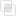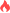python如何将字符转换为数字

Python 字符 转换2021-04-08 11:59:04112948浏览 · 0收藏 · 0评论python中的字符数字之间的转换函数

```int(x [,base ])         将x转换为一个整数
long(x [,base ])        将x转换为一个长整数
float(x )             将x转换到一个浮点数
complex(real [,imag ])     创建一个复数
str(x )              将对象 x 转换为字符串
repr(x )             将对象 x 转换为表达式字符串
eval(str )            用来计算在字符串中的有效Python表达式,并返回一个对象
tuple(s )             将序列 s 转换为一个元组
list(s )             将序列 s 转换为一个列表
chr(x )              将一个整数转换为一个字符
unichr(x )            将一个整数转换为Unicode字符
ord(x )              将一个字符转换为它的整数值
hex(x )              将一个整数转换为一个十六进制字符串
oct(x )              将一个整数转换为一个八进制字符串```

```chr(65)='A'
ord('A')=65

int('2')=2;
str(2)='2'```

python中字符编码是什么？如何转换字符?Python类型转换，数据类型转换函数大全python是如何进行类型转换的python中数据类型如何进行转换python怎么样将字符串转换字符You know nothing!

centos7怎么删除vim10273

centos7中vim怎么配置8822